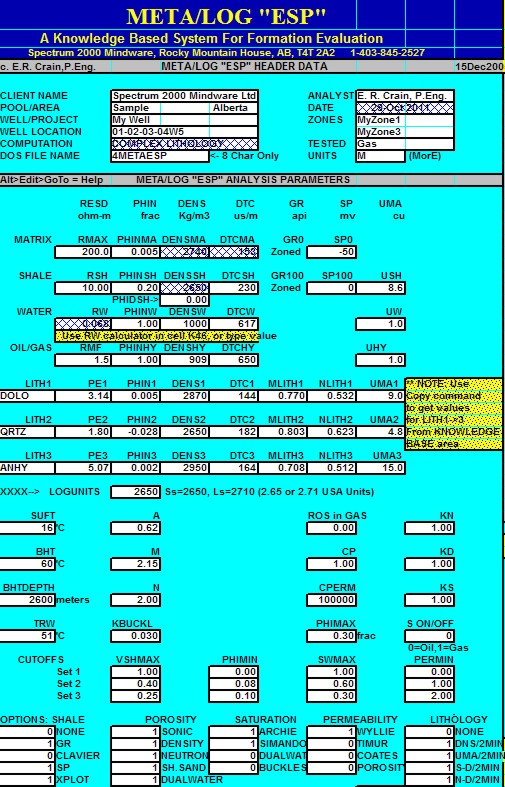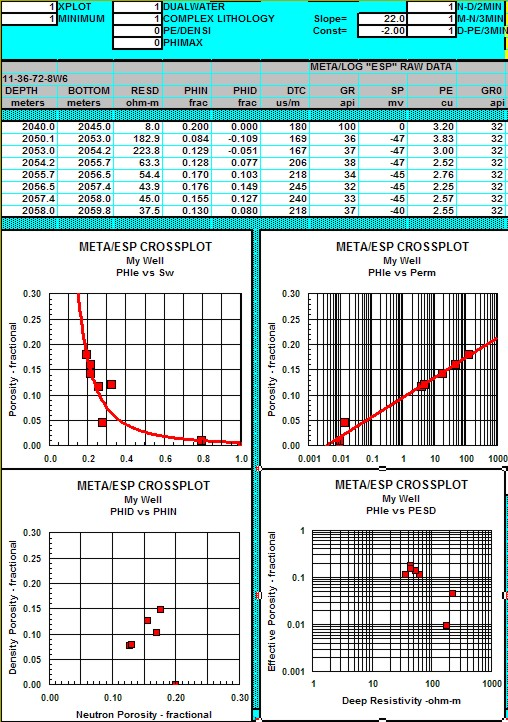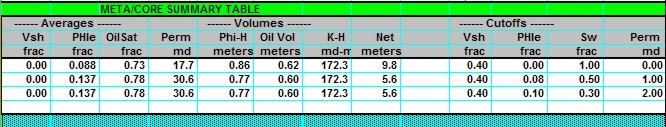Cumulative and Average Reservoir Properties
The reservoir volume and flow capacity per unit area are steps toward finding total reservoir volume. Average values for comparing the quality of reservoirs are also useful results from log analysis. Pore volume (per unit area), hydrocarbon pore volume, flow capacity, and the averages of core porosity, water saturation, permeability, net pay, net reservoir, net sand, and gross sand are called mapable properties, petrophysical properties, or reservoir properties.

References:
1. Interpretation, Averaging and Use of the Basic Geological-Engineering Data
D. Havlena, CIMM/PETSOC, 1966

2. Dynamic Reservoir Data: Part II – Interpretation, Averaging and Use of the
Basic Geological-Engineering Data
.
D. Havlena, CIMM/PETSOC, 1968Cumulative Reservoir Properties
Pore volume (PV).
5: PV = SUM (PHIe * THICK * PAYFLAG)

Hydrocarbon pore volume (HPV).
6: HPV = SUM (PHIe * (1 - Sw) * THICK * PAYFLAG)

Flow capacity (KH).
7: KH = SUM (Perm * THICK * PAYFLAG)

Average porosity.
8: PHIavg = PV / Hnet

Average water saturation.
9: SWavg = 1 - (HPV / PV)

Average permeability.
a. Arithmetic average:
10: Kavg = KH / Hnet

b. Geometric average:
11: Kgeo = (PROD (Perm * THICK)) ^ (1 / Ns)

c. Harmonic average:
12: Khar = Hnet / (SUM (1 / (Perm * THICK)))

Where:
Hnet = net pay thickness (ft or m)
HPV = hydrocarbon volume (ft or m per unit area)
Kavg = arithmetic average permeability (md)
Kgeo = geometric average permeability (md)
KH = flow capacity (md-ft or md-m per unit area)
Khar = harmonic average permeability (md)
Ns = number of samples in product
Perm = permeability (md)
PHIavg = average porosity (fractional)
PHIe = effective porosity (fractional)
PV = pore volume (ft or m per unit area)
SWavg = average water saturation (fractional)
THICK = individual layer thickness (ft or m)COMMENTS:
Do not use the following algorithm in thinly laminated shaly sands - see alternate method shown below.

The harmonic average most closely reflects radial flow into a borehole. If equal sample intervals are used, this geometric formula becomes: Kgeo = (PROD (Perm * INCR)) ^ (1 / INCR). where INCR = data digitizing increment. It does not give the same result as the previous version if layer thicknesses are unequal.Sample net pay table with average and cumulative mapable propertiesNUMERICAL EXAMPLE:
1. Assume three layers as follows:
Layer       PHIe      Sw      Perm      THICK (ft)
1           0.10      0.60        10            2
2           0.20      0.50      100            4
3           0.30      0.40    1000            6

Assume all layers pass all cutoffs:
PV = 0.10 * 2 + 0.20 * 4 + 0.30 * 6 = 2.8 ft
HPV = 0.10 * (1 - 0.60) * 2 + 0.20 * (1 - 0.50) * 4 + 0.30 * (1 - 0.40) * 6 = 1.56 ft
KH = 10 * 2 + 100 * 4 + 1000 * 6 = 6420 md-ft
Hnet = 2 + 4 + 6 = 12 ft
PHIavg = 2.8 / 12 = 0.233
SWavg = 1 - 1.56 / 2.8 = 0.443
Kavg = 6420 / 12 = 535 md
Kgeo = (10 * 2 * 100 * 4 * 1000 * 6) ^ (1 / 3) = 363 md
Khar = 12 / (1 / (10 * 2) + 1 / (100 * 4) + 1 / (1000 * 6)) = 228 md

If equal sample intervals are used, (with INCR = 1.0),
Kgeo = 215 mdMETA/LOG ESP SPREADSHEET -- Log Analysis Sums and Averages
This spreadsheet provides a tool for analyzing and summarizing log data in a consistent format. It calculates porosity and permeability averages, suns pore volume, hydrocarbon pore volume, flow capacity, and net pay with user defined cutoffs in a table identical to that created by the META/LOG "CORE"  spreadsheet for log analysis, making it easy to compare log analysis results to core data.

SPR-04 META/LOG ESP Advanced Log Analysis Oil Gas Metric and USA
Conventional Oil, Gas -- shale, porosity, lithology, saturation, permeability, net pay,, productivity, reserves, crossplots.Exanple of META/LOG ESP input data and crossplots. Intermediate calculations are performed offscreen to the right. The Summary Table (shown below0 is also off to the right.Individual Answers and Summary Table from META/LOG ESP log analysis spreadsheet. Compare to core analysis results shown below.Summary Table from "META/COR". Compare values to "META/ESP" log analysis Summary Table shown above.

Page Views ---- Since 01 Jan 2015
Copyright 2023 by Accessible Petrophysics Ltd.
CPH Logo, "CPH", "CPH Gold Member", "CPH Platinum Member", "Crain's Rules", "Meta/Log", "Computer-Ready-Math", "Petro/Fusion Scripts" are Trademarks of the Author